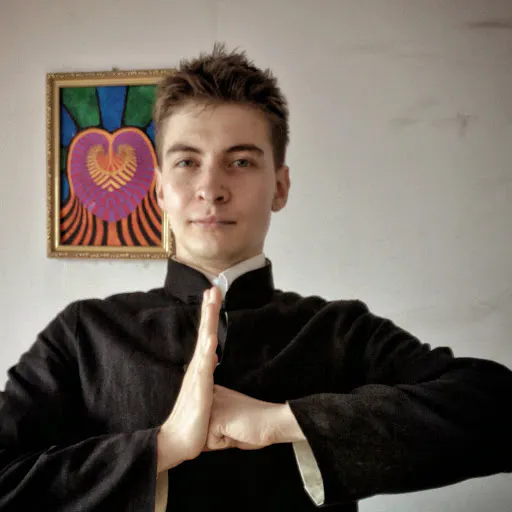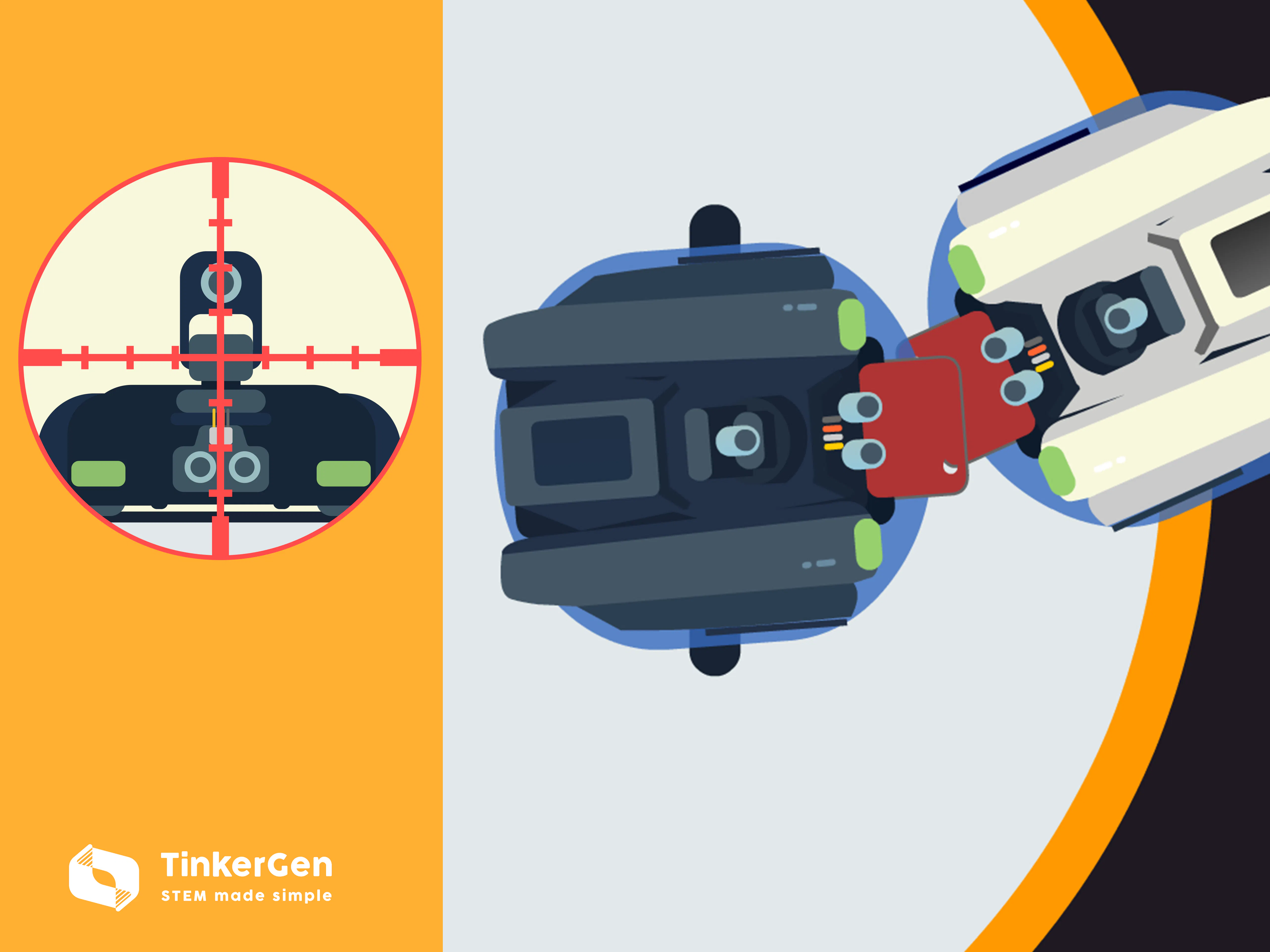# Deep Learning Sumo Robot

Hajimete! Let DL pushing madness begin!

IntermediateProtip3 hours772## Things used in this project

### Hardware components

 Seeed M.A.R.K kit
×1

### Software apps and online services

 aXeleRate

## Code

### MARK micropython sumo code

MicroPython
```import sensor,image,lcd, os, time
from maix_motor import Maix_motor
import KPU as kpu

lcd.init()
sensor.reset()
sensor.set_pixformat(sensor.RGB565)
sensor.set_framesize(sensor.QVGA)
sensor.set_windowing((224, 224))
sensor.set_vflip(1)
sensor.run(1)
DEBUG = 0
classes = ["mark"]
anchor = (0.57273, 0.677385, 1.87446, 2.06253, 3.33843, 5.47434, 7.88282, 3.52778, 9.77052, 9.16828)
a = kpu.init_yolo2(task, 0.6, 0.3, 5, anchor)
while(True):
img = sensor.snapshot().rotation_corr(z_rotation=90.0)
a = img.pix_to_ai()
if code:
for i in code:
a=img.draw_rectangle(i.rect(),color = (0, 255, 0))
a = img.draw_string(i.x(),i.y(), classes[i.classid()], color=(255,0,0), scale=3)
x_center = i.x()+i.w()/2
print(x_center)
if not DEBUG:
if x_center >= 100 and x_center <= 124:
while 1:
Maix_motor.motor_motion(3, 1, 0)
time.sleep(1.5)
Maix_motor.motor_motion(3, 3, 0)
time.sleep(0.1)
Maix_motor.motor_motion(3, 4, 0)
time.sleep(0.1)
if x_center < 100 and x_center > 0: Maix_motor.motor_motion(1, 4, 0)
if x_center > 124: Maix_motor.motor_motion(1, 3, 0)
a = lcd.display(img)
else:
a = lcd.display(img)
if not DEBUG: Maix_motor.motor_motion(1, 3, 0)
```

### OpenCV blob detection for annotations

Python
```# Standard imports
import cv2
import numpy as np;
import os
from pascal_voc_writer import Writer

def create_ann(filename, boundRect):
writer = Writer(os.path.join('Mark',filename), 3000, 4000)
name = filename.split('.')
writer.save('ann/'+name+'.xml')

for file in os.listdir("Mark"):
print(file)
R,G,B = cv2.split(img)

#Rfilter = cv2.bilateralFilter(G,25,25,10)

gray = cv2.cvtColor(img, cv2.COLOR_BGR2GRAY)

ret, thresh = cv2.threshold(gray,50,255,cv2.THRESH_BINARY_INV)

contours, hierarchy = cv2.findContours(thresh,cv2.RETR_EXTERNAL,cv2.CHAIN_APPROX_NONE)

finalImage = cv2.drawContours(img, contours, -1,(0,0,255),3)

maxContour = 0
for contour in contours:
contourSize = cv2.contourArea(contour)
if contourSize > maxContour:
maxContour = contourSize
maxContourData = contour

contours_poly = cv2.approxPolyDP(maxContourData, 3, True)
boundRect = cv2.boundingRect(contours_poly)
print(boundRect)

# Create a mask from the largest contour

# Use mask to crop data from original image
finalImage = np.zeros_like(img)

print(finalImage.shape)
cv2.rectangle(finalImage, (int(boundRect), int(boundRect)), (int(boundRect+boundRect), int(boundRect+boundRect)), (0,255,0),3)

finalImage = cv2.resize(finalImage,(640,480))

cv2.imshow('final',finalImage)
cv2.waitKey(50)
create_ann(file, boundRect)
cv2.destroyAllWindows()
```

## Credits

### Dmitry Maslov

23 projects • 80 followers